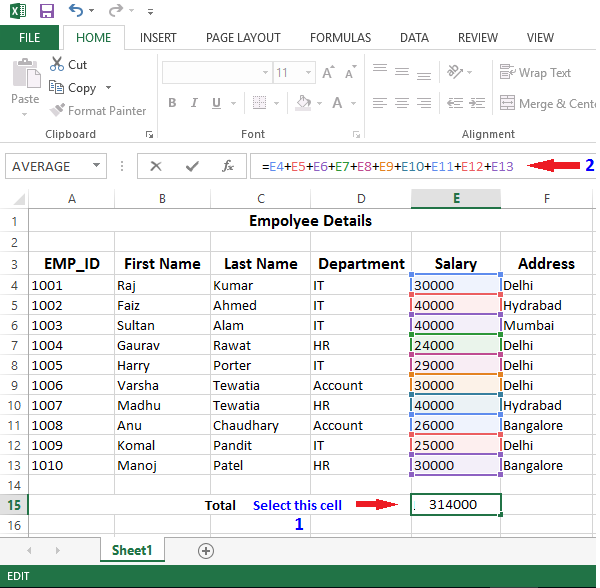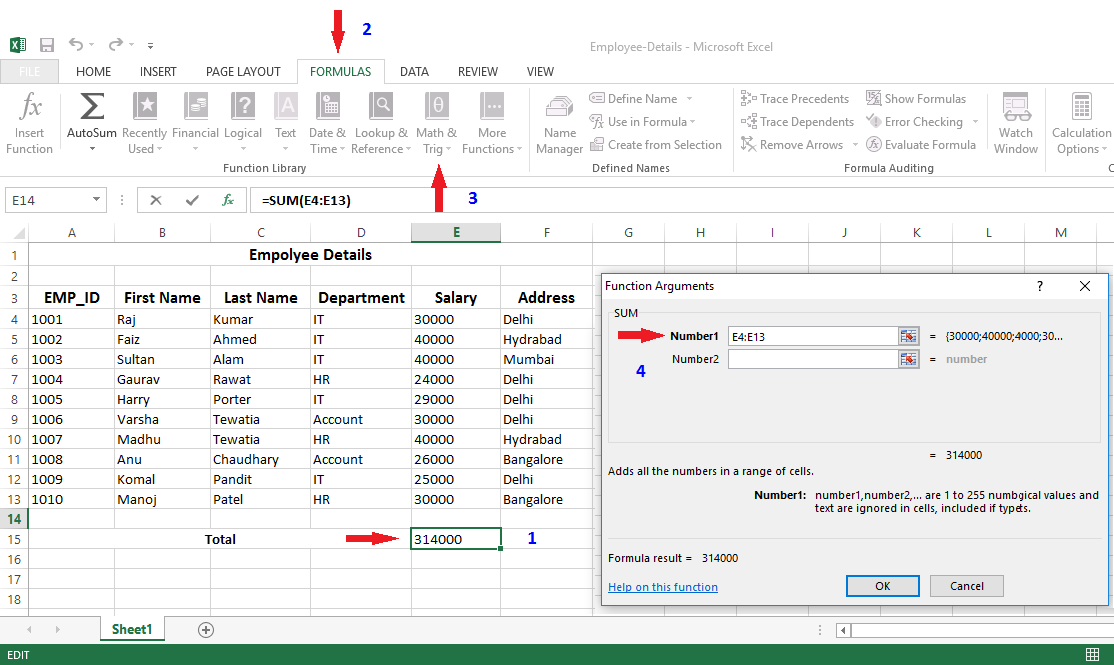# How to do Addition in Excel

## How to Add Data in Excel

There are two way to add column data in excel first is create foumula and other is direct use pre-defined formulas.

### Create Formula

Choose the cell where you want the result to appear as cell E15 in this example. Then type = sign in the cell, now select first cell E4 then type "+" sign then select second cell E5, again type + and select third cell E6 and so on. Now press the enter key, you will get the sum of these salary.### Direct Use Pre-Defined Formulas

• Choose the cell where you want the result to appear as cell E15 in this example.
• Select the Formula tab, then click the Math command.
• Drop down menu will apear now choose SUM
• You can see E4:E14 in this example.